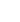# CS 231 Assignment 3CS 231 Programming Assignments# CSC 231

Assignment 3
Due: October 27, Midnight
70 Pts.

Extend the expression simplification presented in class. You are to add the following two simplifications.

First, you must combine all the constants in an expression into a single constant by applying the proper operation. This final constant should be placed in the front of the list. The other parts of the expression should remain unchanged and in their same relative position. For instance, the expression `(+ y 5 x 2 10)` becomes `(+ 17 y x)`. Likewise, `(* 4 3 a (+ 3 y) 2)` becomes `(* 24 a (+ 3 y))`. Note that a single constant is moved to the front of the list: `(+ x 3 y)` becomes `(+ 3 x y)`. Apply this transformation from `simpl` after running flatten. Since you must apply it to both sums and products, you will have to add an argument to `simpl` to which you will pass either the function `'+` or `'*`. You can use this with `reduce` to do the needed arithmetic.

Note that when we combine all the constants, we are not including constants which are not at the top level. For instance, in `(+ 5 (* 4 x) 6)`, we combine 5 and 6, but not the 4. The 4 is part of a multiplication, so it is not considered a "constant" for this purpose. Hence, the simplification produces `(+ 11 (* 4 x))`. Within subexpressions, constants will be combined by the recursion that already exists in `simpl`.

Secondly, within sums, combine identical sub-expressions (other than constants) into a multiplication. Leave the multiplication in place of the first of the repeated items. For instance, `(+ 3 x (* 2 z) y 3 x x t)` becomes `(+ 3 (* 3 x) (* 2 z) y 3 t)`. When the transform creates a multiplication, it should run `flatten` on it, then call the constant combiner mentioned above. So, `(+ (* 2 x) y y (* 2 x) z (* 2 x))` becomes `(+ (* 6 x) (* 2 y) z)`.

Requirement

You must use the functions `remove-if` and `reduce` at least once each.

The constant combination can be written without recursion. Use `remove-if` to separate the make one lists of constants and one list of other arguments. Use `reduce` to combine the list of constants, then `cons` the result back on the front of the other list.
The second one is a bit longer. I created a function called `collect-like-first` which takes a list of arguments and searches for any members which match the first one. If it finds none, it simply returns the original list. Otherwise, it uses `remove-if` to eliminate the duplicates, then adds the multiplication and calls `flatten` and `const-combine`.
I then built a second function that uses a simple recursion to repeatedly run `collect-like-first` on the each member of the list. I made my recursive call with the `cdr` of the list returned from `collect-like-first`.
Both of these transforms will be called from `simpl`. `Simpl` must be modified to call the second one only in case when processing an add. This may require an additional parameter, and will certainly make it less elegant.